# class 7 Maths Chapter-5 line and angles

### Exercise-5.1

Question 1:
Find the complement of each of the following angles: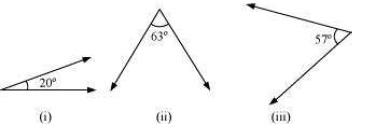The sum of the measures of complementary angles is 90º.
(i) 20°
Complement = 90° − 20°
= 70°
(ii) 63°
Complement = 90° − 63°
= 27°
(iii) 57°
Complement = 90° − 57°
= 33°

Question 2:
Find the supplement of each of the following angles: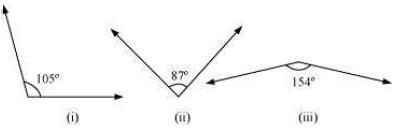The sum of the measures of supplementary anglesis 180º.
(i) 105°
Supplement = 180° − 105°
= 75°
(ii) 87°
Supplement = 180° − 87°
= 93°
(iii) 154°
Supplement = 180° − 154°
= 26°

Question 3:
Identify which of the following pairs of angles are complementary and which are supplementary.
(i) 65°, 115° (ii) 63°, 27°
(iii) 112°, 68° (iv) 130°, 50°
(v) 45°, 45° (vi) 80°, 10°
The sum of the measures of complementary angles is 90º and that of supplementary anglesis 180º.
(i) 65°, 115°
Sum of the measures of these angles = 65º + 115º = 180°
∴ These angles are supplementary angles.
(ii) 63°, 27°
Sum of the measures of these angles = 63º + 27º = 90°
∴ These angles are complementary angles.
(iii) 112°, 68°
Sum of the measures of these angles = 112º + 68º = 180°
∴ These angles are supplementary angles.
(iv) 130°, 50°
Sum of the measures of these angles = 130º + 50º = 180°
∴ These angles are supplementary angles.
(v) 45°, 45°
Sum of the measures of these angles = 45º + 45º = 90°
∴ These angles are complementary angles.
(vi) 80°, 10°
Sum of the measures of these angles = 80º + 10º = 90°
∴ These angles are complementary angles.

Question 4:
Find the angle which is equal to its complement.
Let the angle be x.
Complement of this angle is also x.
The sum of the measures of a complementary angle pair is 90º.
∴ x + x = 90°
2x = 90°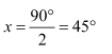Question 5:
Find the angle which is equal to its supplement.
Let the angle be x.
Supplement of this angle is also x.
The sum of the measures of a supplementary angle pair is 180º.
∴ x + x = 180°
2x = 180°
x = 90°

Question 6:
In the given figure, ∠1 and ∠2 are supplementary angles. If ∠1 is decreased, what changes should take place in ∠2 so that both the angles still remain supplementary.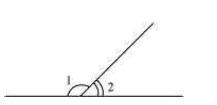∠1 and ∠2 are supplementary angles.
If ∠1 is reduced, then ∠2 should be increased by the same measure so that this angle
pair remains supplementary.

Question 7:
Can two angles be supplementary if both of them are:
(i) Acute? (ii) Obtuse? (iii) Right?
(i) No. Acute angle is always lesser than 90º. It can be observed that two angles, even of 89º, cannot add up to 180º. Therefore, two acute angles cannot be in a supplementary angle pair.
(ii) No. Obtuse angle is always greater than 90º. It can be observed that two angles, even of 91º, will always add up to more than 180º. Therefore, two obtuse angles cannot be in a supplementary angle pair.
(iii) Yes. Right angles are of 90º and 90º + 90º = 180°
Therefore, two right angles form a supplementary angle pair together.

Question 8:
An angle is greater than 45°. Is its complementary angle greater than 45° or equal to 45° or less than 45°?
Let A and B are two angles making a complementary angle pair and A is greater than 45º.
A + B = 90º
B = 90º − A
Therefore, B will be lesser than 45º.

Question 9:
(i) Is ∠1 adjacent to ∠2?
(ii) Is ∠AOC adjacent to ∠AOE?
(iii) Do ∠COE and ∠EOD form a linear pair?
(iv) Are ∠BOD and ∠DOA supplementary?
(v) Is ∠1 vertically opposite to ∠4?
(vi) What is the vertically opposite angle of ∠5?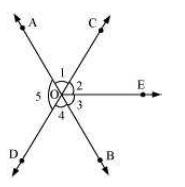(i) Yes. Since they have a common vertex O and also a common arm OC. Also, their non-common arms, OA and OE, are on either side of the common arm.
(ii) No. They have a common vertex O and also a common arm OA. However, their noncommon arms, OC and OE, are on the same side of the common arm. Therefore, these are not adjacent to each other.
(iii) Yes. Since they have a common vertex O and a common arm OE. Also, their noncommon arms, OC and OD, are opposite rays.
(iv) Yes. Since ∠BOD and ∠DOA have a common vertex O and their non-common arms are opposite to each other.
(v) Yes. Since these are formed due to the intersection of two straight lines (AB and CD).
(vi) ∠COB is the vertically opposite angle of ∠5 as these are formed due to the intersection of two straight lines, AB and CD.

Question 10:
Indicate which pairs of angles are:
(i) Vertically opposite angles. (ii) Linear pairs.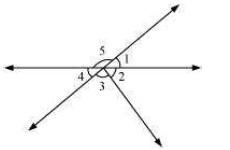(i) ∠1 and ∠4, ∠5 and ∠2 +∠3 are vertically opposite angles as these are formed due to the intersection of two straight lines.
(ii) ∠1 and ∠5, ∠5 and ∠4 as these have a common vertex and also have non-common arms opposite to each other.

Question 11:
In the following figure, is ∠1 adjacent to ∠2? Give reasons.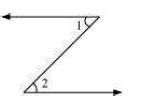∠1 and ∠2 are not adjacent angles because their vertex is not common.

Question 12:
Find the value of the angles x, y, and z in each of the following: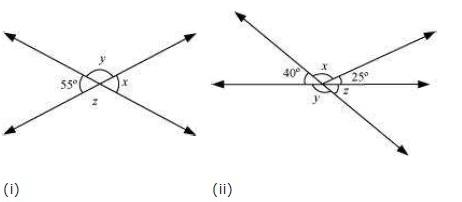(i) Since ∠x and ∠55° are vertically opposite angles,
∠x = 55°
∠x + ∠y = 180° (Linear pair)
55° + ∠y = 180°
∠y = 180º − 55º = 125°
∠y = ∠z (Vertically opposite angles)
∠z = 125°
(ii) ∠z = 40° (Vertically opposite angles)
∠y + ∠z = 180° (Linear pair)
∠y = 180° − 40° = 140°
40° + ∠x + 25° = 180° (Angles on a straight line)
65° + ∠x = 180°
∠x = 180° − 65° = 115°

Question 13:
Fill in the blanks:
(i) If two angles are complementary, then the sum of their measures is _______.
(ii) If two angles are supplementary, then the sum of their measures is _______.
(iii) Two angles forming a linear pair are _______.
(iv) If two adjacent angles are supplementary, they form a _______.
(v) If two lines intersect at a point, then the vertically opposite angles are always _______.
(vi) If two lines intersect at a point, and if one pair of vertically opposite angles are acute angles, then the other pair of vertically opposite angles are _______.
(i) 90°
(ii) 180°
(iii) Supplementary
(iv) Linear pair
(v) Equal
(vi) Obtuse angles

Question 14:
In the adjoining figure, name the following pairs of angles.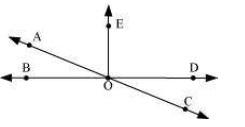(i) Obtuse vertically opposite angles
(iii) Equal supplementary angles
(iv) Unequal supplementary angles
(v) Adjacent angles that do not form a linear pair
(i) ∠AOD, ∠BOC
(ii) ∠EOA, ∠AOB
(iii) ∠EOB, ∠EOD
(iv) ∠EOA, ∠EOC
(v) ∠AOB and ∠AOE, ∠AOE and ∠EOD, ∠EOD and ∠COD

### Exercise-5.2

Question 1:
State the property that is used in each of the following statements?
(i) If a||b, then ∠1 = ∠5
(ii) If ∠4 = ∠6, then a||b
(iii) If ∠4 + ∠5 = 180°, then a||b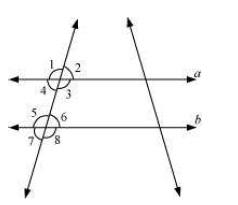(i) Corresponding angles property
(ii) Alternate interior angles property
(iii) Interior angles on the same side of transversal are supplementary.

Question 2:
(i) The pairs of corresponding angles
(ii) The pairs of alternate interior angles
(iii) The pairs of interior angles on the same side of the transversal
(iv) The vertically opposite angles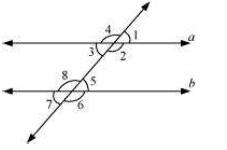(i) ∠1 and ∠5, ∠2 and ∠6, ∠3 and ∠7, ∠4 and ∠8
(ii) ∠2 and ∠8, ∠3 and ∠5
(iii) ∠2 and ∠5, ∠3 and ∠8
(iv) ∠1 and ∠3, ∠2 and ∠4, ∠5 and ∠7, ∠6 and ∠8

Question 3:
In the adjoining figure, p || q. Find the unknown angles.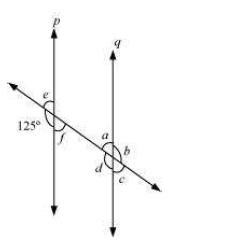∠d = 125° (Corresponding angles)
∠e = 180° − 125° = 55° (Linear pair)
∠f = ∠e = 55° (Vertically opposite angles)
∠c = ∠f = 55° (Corresponding angles)
∠a = ∠e = 55° (Corresponding angles)
∠b = ∠d = 125° (Vertically opposite angles)

Question 4:
Find the value of x in each of the following figures if l || m.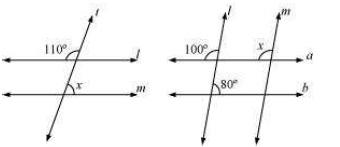(i)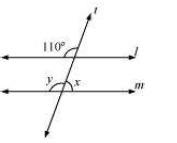∠y = 110° (Corresponding angles)
∠x + ∠y = 180° (Linear pair)
∠y = 180° − 110°
= 70°
(ii)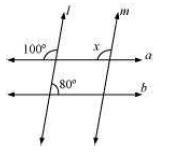∠x = 100° (Corresponding angles)

Question 5:
In the given figure, the arms of two angles are parallel.
If ∠ABC = 70°, then find
(i) ∠DGC
(ii) ∠DEF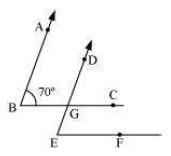(i) Consider that AB|| DG and a transversal line BC is intersecting them.
∠DGC = ∠ABC (Corresponding angles)
∠DGC = 70°
(ii) Consider that BC|| EF and a transversal line DE is intersecting them.
∠DEF = ∠DGC (Corresponding angles)
∠DEF = 70°

Question 6:
In the given figures below, decide whether l is parallel to m.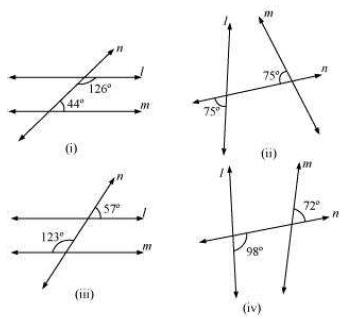(i)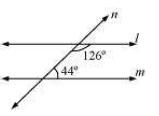Consider two lines, l and m, and a transversal line n which is intersecting them. Sum of the interior angles on the same side of transversal = 126º + 44º = 170° As the sum of interior angles on the same side of transversal is not 180º, therefore, l is not parallel to m.
(ii)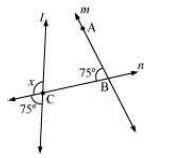x + 75° = 180° (Linear pair on line l)
x = 180° − 75° = 105°
For l and m to be parallel to each other, corresponding angles (∠ABC and ∠x)should be equal. However, here their measures are 75º and 105º respectively. Hence, these lines are not parallel to each other.
(iii)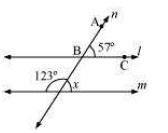∠x + 123° = 180° (Linear pair)
∠x = 180° − 123º = 57°
For l and m to be parallel to each other, corresponding angles (∠ABC and ∠x)should be equal. Here, their measures are 57º and 57º respectively. Hence, these lines are parallel to each other.
iv.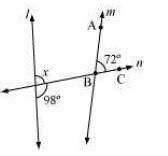98 + ∠x = 180° (Linear pair)
∠x = 82°
For l and m to be parallel to each other, corresponding angles (∠ABC and ∠x)should be equal. However, here their measures are 72º and 82º respectively. Hence, these lines are not parallel to each other.

Get 30% off your first purchase!

X
error: Content is protected !!
Scroll to Top